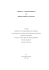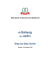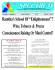# Blind Timing Skew Estimation Using Source Spectrum Sparsity in

## Transcription

Blind Timing Skew Estimation Using Source Spectrum Sparsity in
```IEEE TRANSACTIONS ON INSTRUMENTATION AND MEASUREMENT, VOL. 61, NO. 9, SEPTEMBER 2012
2401
Blind Timing Skew Estimation Using Source
Yue Xian Zou, Senior Member, IEEE, and Xiang Jun Xu
Abstract—Timing skews in time-interleaved analog-to-digital
dynamic range of the TIADC, which can be improved effectively
by digital compensation algorithms. Literature review shows
that many nonblind compensation algorithms required the accurate timing skew parameters measured before the compensation
process. In this paper, the blind timing skew estimation (TSE) for
the TIADC system is investigated. With the evaluation of the working rationale of the TIADC system and the undersampling nature
in each sub-ADC, the relation between the output digital spectra
of the sub-ADCs and the source analog spectrum is formulated.
A novel subband partition approach has been proposed, and the
corresponding expression of the output digital subband spectra of
the sub-ADCs in terms of the output digital subband spectra of
the TIADC is obtained. With the assumption of the source spectra
sparsity, the nonoverlapping frequency points can be determined
from the output digital subband spectra of the sub-ADCs. Making
use of the determined nonoverlapping frequency points, the phase
information of the output digital subband spectra of the sub-ADCs
can be employed to provide a good metric to estimate the timing skews, and a closed-form expression of the TSE has been
derived. As the result, the source spectrum sparsity-based blind
TSE (SS-BLTSE) algorithm has been developed and evaluated.
Computer simulation results show that the SS-BLTSE algorithm
provides good TSE performance for arbitrarily narrow or wideband source signals. Moreover, it has many merits over the existing
blind TSE algorithms, such as there is no prior requirement of the
source signal except that it has certain spectrum sparsity, no limit
to the number of the sub-ADCs, and the frequency of the input
signal can be approaching the Nyquist frequency.
skews, undersampling.
I. I NTRODUCTION
A
Fig. 1. Conceptual illustration of TIADC and timing mismatches with M =
4. (a) Conceptual illustration of M -channel TIADCs. (b) Illustration of the
timing skew effect in TIADC output spectra.
Manuscript received September 13, 2011; revised January 29, 2012; accepted
February 24, 2012. Date of publication May 3, 2012; date of current version
August 10, 2012. This work was supported in part by the National Natural
Science Foundation of China under Grant 60775003 and in part by the Shenzhen Science and Technology Program. The Associate Editor coordinating the
review process for this paper was Dr. Rik Pintelon.
The authors are with the Advanced Digital Signal Processing Laboratory,
Shenzhen Graduate School, Peking University, Shenzhen 518055, China
(e-mail: [email protected]; [email protected]).
Color versions of one or more of the figures in this paper are available online
at http://ieeexplore.ieee.org.
Digital Object Identifier 10.1109/TIM.2012.2192337
interleaved technique proposed by Black and Hodges  that
In principle, the TIADC is formed by using M identical
rate of fs /M as shown in Fig. 1(a). As the result, an overall
sampling rate of fs can be achieved. Each channel samples the
input signal xa (t) in turn, and the outputs are combined into one
discrete output signal y(n). Ideally, all the M parallel channels
are assumed to be linear and identical. They should have
the same gain (gi = constant, i = 0, . . . , M − 1) and should
be operating at the precise equally displaced sampling time
instants. However, due to the practical implementation constraints in the fabrication process, the TIADC system exhibits
HIGH-speed and high-resolution analog-to-digital converter (ADC) is a key component for many modern
electronic systems such as radars, communication systems,
and medical instruments. For a given fabrication technology
and ADC word length requirement, there is a limit to the
maximum achievable sampling speed. To achieve a conversion
speed higher than that achievable by a single ADC, the time-
2402
IEEE TRANSACTIONS ON INSTRUMENTATION AND MEASUREMENT, VOL. 61, NO. 9, SEPTEMBER 2012
the “channel gain mismatch,” “channel bandwidth mismatch,”
“channel dc offset mismatch,” and the “channel timing skew
mismatch” . For illustration purpose, the effect of timing
skew mismatch is shown in Fig. 1(b) with a sinusoidal input
signal. It is noted that, in Fig. 1(b), three distortion components caused by the timing skew mismatch severely reduce the
spurious-free dynamic range of the TIADC . More detailed
discussions on mismatches can be found in –.
The digital compensation of TIADC channel mismatches has
attracted great attention recently. It is noted that several authors
have proposed blind equalization techniques , . Blind
techniques do not require using a known signal to estimate the
channel mismatch parameters and so do not suffer from “downtime problem,” but blind techniques use the excess bandwidth
as a result of oversampling to estimate the mismatch parameter
online. Experimental results showed that the blind equalization
techniques, in general, asked for extra hardware (such as more
ADCs and low-pass filter ). However, their performance of
the timing mismatch compensation is inferior to that of the
nonblind mismatch compensation techniques. In contrast to the
blind techniques, many nonblind timing mismatch compensation algorithms have been proposed –, where the channel timing mismatch parameters are needed. In , Johansson
and Löwenborg cascaded fractional delay filters to compensate
the timing mismatch. In , a multichannel-filtering approach
is used for the TIADC mismatch compensation, which is computationally less complex and more robust than the filter-bank
approach. Recently, Zou et al. introduced a novel multichannel
Lagrange polynomial interpolation-timing mismatch compensation algorithm , which leads to a real-time implementation filter structure with low computational complexity.
Careful evaluation reveals that these nonblind compensation
methods all ask for the good measurement of the channel mismatch parameters. However, the measurement of the channel
mismatch parameters using either online or offline approaches
is a very challenging task –. In principle, the offline
approaches need to know the input calibration signal. On the
contrary, the blind parameter estimation methods only request
statistical information on the input signal in an online manner,
which has the advantages of being able to track the channel
mismatch varying without interruption of the operation of
the TIADC system. The blind timing skew estimation (TSE)
has attracted many researchers working on it from different
perspectives , –. Jamal et al.  and Huang and
Levy  employed an oversampling technique and used the
alias-free bandwidth to estimate the channel mismatch parameters in a blind estimation manner. Unfortunately, these methods
presented poor scalability. Zou et al.  proposed an efficient
blind TSE method for the TIADC systems by evaluating the
difference between the output samples of the two adjacent subADCs. However, this approach only works well when the frequency bandwidth of the analog input signal is lower than half
of the Nyquist frequency. In , Elbornsson et al. integrated
the channel mismatch parameter estimation and compensation
into a uniform framework known as blind adaptive equalization.
The mismatches are compensated using their proposed iterative
the signal continuity, and it works well for signals whose energy
concentrates in low-frequency bands.
In this paper, we focus on the blind TSE problem of the
TIADC systems, where the channel gain mismatch and dc
offset mismatch are not taken into account, although it has been
shown that either gain mismatch or dc offset mismatch will
have no impact on the performance of our proposed algorithm.
In one word, our task is to estimate the channel timing skews
Δtm (m = 0, 1, . . . , M − 1) in a blind manner. With careful
evaluation of the working rationale of the TIADC system
and the undersampling nature in each sub-ADC, we are able
to determine some nonoverlapping frequency points from the
output spectra of the TIADC system when the input analog
signal has a certain frequency spectrum sparsity attribute. With
the determined nonoverlapping frequency points, it is found
that the phase information of the spectra can be employed to
provide a good metric to estimate the timing skews.
For getting the tractable solution, we have made the following assumptions.
1) Input signal xa (t) is bandlimited with the highest frequency smaller than fs /2.
2) The system parameters of the TIADC system are known,
such as the number of sub-ADCs (M ) and the overall
sampling frequency (fs ).
3) The discrete output signals (ym (n), y(n)) are available.
4) No channel dc offset and gain mismatches are considered
since they can be compensated using the simple subtraction and scalar gain approaches .
5) No additive noise is considered.
6) As the timing skew is usually much smaller than the
sampling period (smaller than 10%), the input analog
signal is much stronger than the disturbance introduced
by TIADC channel timing skews .
Literature review shows that the aforementioned assumptions
are commonly satisfied in the research of the TIADC technology , .
This paper is organized as follows. The formulation of the
digital spectra of the ideal TIADC system is presented in
Section II. The spectrum sparsity-based blind TSE (SS-BLTSE)
algorithm is derived in detail and summarized in Section III.
Computer simulation results are presented in Section IV.
Section V gives the conclusions of our work.
II. D IGITAL S PECTRA M ODELING FOR I DEAL TIADC
In this section, we will present the formulation of the output
system, in which no channel mismatches, additive thermal
noise, and quantization error are considered.
A. Digital Spectra Representation for the Ideal TIADC and
In this paper, the architecture of the TIADC considered
is illustrated in Fig. 1(a), where the analog input xa (t) is a
bandlimited signal with the highest frequency of fmax (Hz).
The analog spectrum (Fourier transform) of xa (t) is denoted as
Xa (jΩ) , where Ω = 2πf is the analog radian frequency in
ZOU AND XU: BLIND TSE USING SOURCE SPECTRUM SPARSITY IN TIME-INTERLEAVED ADCs
radians per second and f is the analog frequency in cycles per
second (in hertz).
The output discrete-time signal of the ideal ADC with the
sampling period of Ts can be denoted as
xd (n) = xa (t)|t=nTs ,
n = 0, 1, . . . , ∞.
(1)
The digital spectrum [the discrete-time Fourier transform
(DTFT)] of xd (n) is denoted as Xd (ejω ) , where ω is
the digital frequency in radians per sample associated with the
sampling frequency of fs , which has the following relation:
ω = ΩTs = 2πf /fs
(2)
where fs is the sampling frequency and fs = 1/Ts . Therefore,
the relation between the analog and the digital spectrum can be
denoted as 
+∞
ω
1 2πk
Xd (e ) =
Xa j
−
.
Ts
Ts
Ts
jω
(3)
2403
where Tss is the sampling period of the sub-ADCs, N is the
total number of samples for TIADC, and m is the channel
index.
Let us define the digital frequency associated with the sampling period of Tss as , which has the following relation
with the analog frequency Ω associated with the sampling
frequency fss :
= ΩTss = 2πf /fss .
(7)
From (6) and (7), the DTFT of ym (n) can be expressed as
∞
2πk
2πk
1 j
Ym (e ) =
Xa j
−
ej ( Tss − Tss ) mTs .
Tss
Tss Tss
k=−∞
(8)
It can be seen that Ym (ej ) is also a summation of the
frequency-scaled and the shifted version of Xa (jΩ), but the
digital frequency is specified in (7). Obviously, Ym (ej ) is also
a periodic function of with a period of 2π.
k=−∞
From (3), it is noted that the digital spectrum Xd (ejω ) is a
summation of the frequency-scaled and the shifted version of
Xa (jΩ), where the frequency scaling is specified in (2). It is
also a continuous periodic function of ω with a period of 2π.
Since the frequency range of xa (t) is bandlimited to
(−fmax , fmax ), the digital spectrum of xd (n) in its basic band
can be denoted as
⎧
⎨ 1 Xa j ω ,
ω ∈ (0, π)
T
T
s
s (4)
Xdp (ejω ) =
1
ω−2π
⎩ Xa j
,
ω ∈ (π, 2π)
Ts
Ts
where Xdp (ejω ) specifically represents the principal component of Xd (ejω ). According to the Nyquist sampling theorem,
the analog signal xa (t) can be perfectly reconstructed from its
ideal sampling sequence xd (n).
From the TIADC system shown in Fig. 1(a), in the ideal case,
the output of the TIADC will be identical to its counterpart of
y(n) = xd (n).
(5)
It is noted that the overall sampling frequency of an ideal
M -channel TIADC system is the same as that of an ideal ADC
system. Hence, it is also denoted as fs , while the sampling frequency for each subchannel (sub-ADC) in the TIADC system
denoted as fss turns to be fss = fs /M .
In the following context, we will derive the relation between
the output digital spectra of the sub-ADCs and the input analog spectrum using the TIADC system architecture shown in
Fig. 1(a).
From Fig. 1(a), it is straightforward to get the time domain
expression of the output of the mth sub-ADC
ym (n) = xa (t)|t=nTss +mTs ,
n = 0, 1, . . . , N/M − 1,
Tss = 1/fss ,
m = 0, 1, . . . , M − 1 (6)
B. Digital Frequency Subband Modeling in Ideal
From the working principle of the TIADC system, we have
the following observations: 1) If xa (t) is bandlimited with the
upper bound of fs /2, the xa (t) can be reconstructed perfectly
from the output xd (n) of the ideal TIADC, and 2) for each
sub-ADC in TIADC system, it operates in undersampling situation with an undersampling factor of M . In what follows,
the relation between xa (t) and ym (n) for an ideal TIADC
system will be explored in the time domain and spectrum
domain. A new spectrum partition approach is proposed, and
the relation between the partitioned spectra of the sub-ADCs
and the partitioned spectra of the TIADC has been derived.
As discussed earlier, the analog frequency Ω and the digital
frequency ω is interconnected by the sampling frequency, such
as specified in (2) or (7). Let us partition the analog-frequency
range (−Ωs /2, Ωs /2) into 2M equal bandwidth bands, where
Ωs = 2πfs is the sampling angular frequency of the TIADC
system. We name each band as one analog-frequency subband
(AFSB). The bandwidth of each AFSB is given by
Δfa = Ωs /2/M = πfs /M.
(9)
Therefore, the principle digital frequency ranging from 0 ∼
2π correspondingly can be partitioned into 2M digital bands
with equal bandwidth, which is defined as one digital-frequency
subband (DFSB) correspondingly. It is obvious that the bandwidth of a DFSB associated with the sampling period of Ts can
be determined as
Δfd = Δfa × Ts =
π
.
M
(10)
Similarly, the bandwidth of a DFSB associated with the
sampling period of Tss can be determined as
Δfm = Δfa × Tss = π.
(11)
2404
IEEE TRANSACTIONS ON INSTRUMENTATION AND MEASUREMENT, VOL. 61, NO. 9, SEPTEMBER 2012
As the result, the principle digital spectrum of xd (n) can be
partitioned into 2M DFSBs with a bandwidth of Δfd in (10);
meanwhile, the principle digital spectrum of ym (n) only can be
partitioned into two DFSBs with a bandwidth of Δfm in (11).
Specifically, let us define the kth component of Xdp (ejω ) in
(4) at the kth DFSB as follows:
(k−1)π kπ
jω
(k) jω
X
(e
)
ω
∈
,
dp
M
M , k = 1, 2, . . . , M
Xdp (e ) =
0
others.
(12)
Similarly, the first and second
Ym (ej ) in (8) are given by
j
(1) j
Ym (e ) = Ym (e )
0
j
Ym(2) (ej ) = Ym (e )
0
DFSB components of
∈ (0, π)
others
∈ (π, 2π)
others.
(13)
Next, we will investigate the relations between Xdp (ejω ) and
(2)
and Ym (ej ).
Here, we define ω2π = ((ω + π) mod 2π) − π. According
to assumption 1), Xa (jΩ) = 0 for Ω > |2πfs |, and (8) can be
rewritten as follows:
(1)
Ym (ej )
M
j
Ym (e
1
)=
Tss
2
k=−M/2=1
2πk
Xa j
−
Tss
Tss
×ej ( Tss − Tss )mT ,
2πk
∈ (0, 2π).
(14)
Replacing Xa (jΩ) by Xdp (ejω ) using the relation expressed
in (4), together with Tss = M ∗ Ts , (14) can be derived as
follows:
1
Ym (ej ) =
M
0
Xdp ej (
M
− 2πk
M
) ej (
in (13) can be rewritten as follows:
M
− 2πk
M
)m .
k=−M +1
(15)
With variable exchange, (15) can be equivalently rewritten as
j
Ym (e
M −1
2πk 2πk
1 )=
Xdp ej ( M + M ) ej ( M + M )m
M
k=0
2π(k−1) M
2π(k−1)
1 j M
+
j
+
m
M
M
=
Xdp e
.
e M
M
k=1
(16)
It is noted that, if ∈ (0, π), we have ((/M ) +
(2(k−1)π/M )) ∈ ((2(k−1)π/M ), ((2k−1)π/M )), k = 1,
(1)
2, . . . , M . According to (12) and (16), Ym (ej ) in (13) can
be rewritten as follows:
Ym(1) (ej ) =
M
π
1 (2k−1) j ( M
+2(k−1) M
)
Xdp
e
M
k=1
π
jm( M
+2(k−1) M
)
×e
2π
,
Fig. 2. Conceptual illustration of AFSBs and DFSBs and the corresponding
spectra (M = 4, Ωmax < Ωs /2). (a) |Xa (jΩ)|. (b) |Xd (ejω )| in (0 ∼ 4π).
(c) |Ym (ej )| in (0 ∼ 4π).
∈ (0, π).
(17)
In the same way, if ∈ (π, 2π), then ((/M ) + (2(k − 1)π/
(2)
M )) ∈ ((2k−1)π/M ), (2kπ/M )), k = 1, 2, . . . , M , Ym (ej )
Ym(2) (ej ) =
M
π
1 (2k) j ( M
+2(k−1) M
)
e
Xdp
M
k=1
π
jm( M
+2(k−1) M
)2π
, ∈ (π, 2π).
×e
(18)
To get more understanding of the aforementioned derivation,
an illustration is shown in Fig. 2, where we set M = 4. The
partition of the analog amplitude spectrum (|Xa (jΩ)|) and the
eight equal bandwidth AFSBs ranging from the −3th AFSB
to the 4th AFSB are plotted in Fig. 2(a). The corresponding
partition of |Xd (ejω )| in (0, 2π) and the eight equal bandwidth
DFSBs are illustrated in Fig. 2(b). Correspondingly, the partition of |Ym (ej )| in (0, 2π) and two equal bandwidth DFSBs
are presented in Fig. 2(c). Obviously, from Fig. 2(a), the highest
frequency component is smaller than Ωs /2, so there is no
spectrum overlapping in Xd (ejω ) since its sampling frequency
is Ωs . However, from Fig. 2(c), since the subchannel sampling
frequency is reduced to Ωs /4, we can see that the spectrum
overlapping occurs in Ym (ej ). It is clear to see that there are
still some frequency components that are not overlapped such
as the spectrum line labeled as Ym (ej1 ) in Fig. 2(c). We can
infer that, if the analog spectrum Xa (jΩ) has certain spectrum
sparsity property, which we will discuss in the next section,
most probably, there are certain nonoverlapping frequency
components ωp existing in Ym (ej ) where ωp ∈ (0, 2π).
ZOU AND XU: BLIND TSE USING SOURCE SPECTRUM SPARSITY IN TIME-INTERLEAVED ADCs
III. S PARSE S PECTRUM -BASED B LIND TSE A LGORITHM
In this section, we will derive a blind TSE algorithm jointly
making use of the TIADC system structure and the relation
between the partitioned spectra derived in the previous section.
A. SS-BLTSE Algorithm
Take sub-ADC-0 as the reference channel without lost of
generality. Define the channel relative timing skew Δm as
Δm =
Δtm (tm −tm )
=
, m = 0, 1, . . . , M −1, Δ0 = 0
Ts
Ts
(19)
where Δtm is the timing skew for the mth sub-ADC and tm
and tm are the real and the ideal sampling instant for the mth
sub-ADC, respectively. From (6), ym (n) with timing skews can
be reformulated as
ym (n) = xa (nM Ts +(m+Δm)Ts), n = 0, . . . , N/M −1. (20)
(1)
Correspondingly, from (17) and (18), Ym (ej ) and
(2) j
Ym (e ) with timing skews will have the following form:
M
π
1 (2k−1) j ( M
+2(k−1) M
)
e
Xdp
M
k=1
π
j(m+Δm )( M
+2(k−1) M
)2π
, ∈ (0, π) (21)
×e
M
π
1
(2k)
ej ( M +2(k−1) M )
Ym(2) (ej ) =
Xdp
M
k=1
π
j(m+Δm )( M
+2(k−1) M
)2π
, ∈ (π, 2π). (22)
×e
Ym(1) (ej ) =
Comparing (17) and (21), it is noted that the timing skews
(1)
(2)
only introduce phase change in Ym (ej ) and Ym (ej ).
(1)
Carefully evaluating (21), we observe that Ym (ej ) is the
summation of the frequency-expanded and the shifted version
of Xdp (ejω ) at the (2k − 1)th DFSB in (0, 2π), where k is
(2)
an integer. Meanwhile, Ym (ej ) is the summation of the
frequency-expanded and the shifted version of Xdp (ejω ) at the
2kth DFSB in (0, 2π), where k is an integer. Therefore, if
the signal spectrum Xdp (ejω ) has certain sparse property, we
can infer that there exist some nonoverlapping frequencies
either in (0, π) or (π, 2π). Without loss of generality, here, we
consider the nonoverlapping frequency denoted as ωp , which
exists in (0, π). From (21), for arbitrary ωp , we have
π
1 (2kp −1) j ( ωMp +2(kp −1) M
)
Xdp
Ym(1) (ejωp ) =
e
M
ω
π
j(m+Δm )( Mp +2(kp −1) M
)2π
, ωp ∈ (0, π) (23)
×e
where 2kp − 1 is the DFSB index associated with ωp .
(1)
YM (ejωp )
(1)
Y0 (ejωp )
2405
(1)
(2)
Similarly, the derivation of the Δm using Ym (ej ) gives
(2) jωp
ln Ym(2) (ejωp )
Y0 (e
)
Δm = ωp
−m, ωp ∈ (π, 2π). (26)
π
j M +2(kp −1) M
2π
In the following section, we will introduce a systematic
method to determine the nonoverlapping frequencies ωp and the
related parameter kp . Moreover, it is noted that ωp is computed
by (23) where Xd (ejω ) is assumed to be known. Carefully
evaluating Xd (ejω ) when Δm is much smaller than Ts and with
no gain mismatch and dc offset mismatch considered, according
to assumption 6) in the Introduction, Xd (ejω ) can be approximately estimated by Y (ejω ), which is the DTFT of the output
of the TIADC. According to this derivation, the estimate of Δm
from (25) and (26) can be categorized as a blind TSE algorithm
since it only requires the output spectra of the TIADC system
and has no requirement on the input spectrum. Moreover, we
name this proposed algorithm as an SS-BLTSE algorithm since
it essentially was derived in the frequency domain and with the
spectra sparsity assumption of the analog signal.
B. Estimation of Nonoverlapping Frequencies
in Frequency Domain
In this section, we will introduce a method to determine
the nonoverlapping frequency ωp using the relation between
the spectra. Here, we still consider ωp locals in (0, π). The
(1)
first DFSB spectrum component Ym (ej ) is given in (17)
and illustrated in Fig. 2(c). According to this formulation, we
have the following facts: 1) If ωp ∈ (0, π) is a nonoverlap(1)
(1)
ping frequency, then Ym (ejωp ) = 0, and 2) since Ym (ejωp )
is the summation of the frequency-expanded and the shifted
version of Xdp (ejω )|ω=ωp /M +2π(k−1)/M at the (2k − 1)th
DFSB in (0, 2π), where k is an integer, therefore, for spectra components in the odd DFSBs, there should be only
the corresponding (2kp − 1)th DFSB spectrum component
(2k −1)
Xdp p (ej((ωp /M )+2(kp −1)(π/M )) ) of Xdp (ejω ) that has a
ω
π
ωp
π
j(m+Δm )( Mp +2(kp −1) M
)2π
ej ( M +2(kp −1) M ) e
=
ωp
ω
π
π
jΔ0 ( Mp +2(kp −1) M
(2k −1)
)2π
ej ( M +2(kp −1) M ) e
Xdp p
ω
π
j(m+Δm )( Mp +2(kp −1) M
)2π
ω
π
e
j(m+Δm )( Mp +2(kp −1) M
)2π
=
=
e
ωp
π
jΔ0 ( M +2(kp −1) M
)2π
e
(2kp −1)
(1)
From (23), dividing Ym (ejωp ) by Y0 (ejωp ), (m = 0, 1,
. . . , M − 1), we have (24) shown at the bottom of the page.
The last equation in (24) is obtained by using Δ0 = 0. After
simple manipulation, the closed-form expression of Δm can be
derived as
(1) jωp
ln Ym(1) (ejωp )
Y0 (e
)
Δm = ωp
− m, ωp ∈ (0, π). (25)
π
j M + 2(kp − 1) M
2π
Xdp
(24)
2406
IEEE TRANSACTIONS ON INSTRUMENTATION AND MEASUREMENT, VOL. 61, NO. 9, SEPTEMBER 2012
value, and the other components are zero in the ideal case. The
nonoverlapping frequencies can be determined by Algorithm I
presented hereinafter.
Algorithm I: Determine the nonoverlapping frequency points
Step 1) Scanning the digital frequency ranging from 0 to π,
find the nonoverlapping frequency candidate set by
(1)
(27)
i : f Y0 (eji ) = 0, i = 1, . . . , L1 .
Step 2) For each i obtained in (27), the amplitude frequency spectrum (AFS) is calculated as follows:
π
(2k−1) j ( Mi +2(k−1) M
) , k = 1, . . . , M (28)
e
Nx (k, i) = Xdp
(2k−1)
where 2k − 1 is the DFSB index and Xdp
(ejω )
is approximately estimated by the mismatched
TIADC output Y (ejω ). From (28), for each i , it
is noted that Nx (k, i) is an M × 1 vector. If and
only if there is only one DFSB (the (2kp − 1)th)
that gives Nx (kp , i) = 0 (the additive thermal noise
and quantization error will be considered in the next
section), then i is viewed as a nonoverlapping
frequency candidate, and we denote the AFS of the
jth nonoverlapping frequency as
Np (kp , j) = Nx (kp , i),
ωp (j) = i
(29)
where kp is determined from the DFSB index where
i is taken as a nonoverlapping frequency and ωp (j)
means the jth nonoverlapping frequency candidate
recorded. Repeat the same procedure for all i ’s,
and as the result, we may get the nonoverlapping frequency set {ωp (j), j = 1, . . . , HL , HL ≤ L1 } and its
corresponding AFS set {Np (kp , j), j = 1, . . . , HL }.
Step 3) Find the maximum of the AFS set {Np (kp , j), j =
1, . . . , HL }, and correspondingly, the nonoverlapping frequency ωp1 ∈ (0, π) and its DFSB index can
be determined.
Step 4) For the digital frequency ranging from π to 2π, we
follow the same procedure described in Steps 1)–3)
(2)
(2k)
using |Ym (ej )| and |Xdp (ej((/M )+2(k−1)(π/M )))|,
k = 1, . . . , M , to determine the nonoverlapping
frequency ωp2 ∈ (π, 2π).
C. Estimation of Nonoverlapping Frequencies in Discrete
Frequency Domain
In Section III-B, we have presented the estimation of
nonoverlapping frequencies in the frequency domain systematically, where the nonoverlapping frequency ωp and its related
DFSB index 2kp − 1 is determined from Xdp (ejω ). However,
practically, we use N -point discrete Fourier transform (DFT)
of y(n) to approximately estimate Xdp (ejω ). Essentially,
N -point DFT is the sample version of DTFT, and DFT is
defined in the discrete frequency domain. Regarding the estimation accuracy of ωp , we need to consider two issues: 1) the
spectrum leakage due to N -point DFT and 2) the discrete
nonoverlapping frequency estimation bias due to the DFT.
First, considering the spectrum leakage of the N -point DFT
(although the Hann window can be employed to ease this effect)
and other noises (such as quantization noise and thermal noise),
in general, the estimated Xdp (ejω ) in its DFSBs may not be
zeros for some frequencies, while they are zeros in the ideal
case. Therefore, when the amplitude spectrum at a frequency is
small to some extent, we consider that it is caused by spectrum
leakage and noises, and its effect should be neglected. Hence, it
is reasonable to employ a preprocessing before the selection of
the nonoverlapping frequency ωp , where a soft thresholding is
applied on Xdp (ejω )
Xdp (ejω )=
0
Xdp (ejω )
if |Xdp (ejω )|≤εx max |Xdp (ejω )|
others
(30)
where the predefined threshold parameter εx is related to the
window function used for DFT, signal-to-noise ratio (SNR), and
quantization bits.
Second, since the spectrum of y(n) only can be obtained
by an N -point DFT, which is a discrete frequency spectrum,
the nonoverlapping frequency estimation bias is inevitable. The
larger N will lead to small nonoverlapping frequency estimation bias at the higher computational complexity. A tradeoff between estimation bias and the length of DFT will be taken into
account in practical applications. Simulation results showed
that the estimation accuracy of Algorithm I is acceptable.
D. Analysis of Signal Spectrum Sparsity
In this section, the definition of the spectrum sparsity is
given, and the requirement of the spectrum sparsity for our
proposed SS-BLTSE algorithm will be discussed. With the
definition of the spectrum of Xdp (ejω ), which is approximately
estimated by the output spectrum of TIADC denoted as Y (ejω ),
the spectrum sparsity of the input signal is defined as SSL =
Nk /NT , where Nk denotes the number of the nonzero spectrum
components of Xdp (ejω ) and NT denotes the total number of
the spectrum components of Xdp (ejω ).
As discussed earlier, for our proposed SS-BLTSE algorithm,
it asks for certain signal spectrum sparsity. For making the problem manageable, we define two types of amplitude spectrum of
discrete spectrum. If the amplitude spectrum is the continuous
function over certain positive frequency range Rf (in hertz),
such spectrum is termed as a continuous spectrum defined over
Rf˙ ; if the spectrum does not satisfy the definition of the range
continuous spectrum, it is termed as the discrete spectrum. It
is easy to understand that, if the continuous spectrum defined
on (fx , fx + Rf ) and Rf < fs /2, fs is the sampling rate, then
it is expected that its digital spectrum will exist in (ωx , (ωx +
DRf )) in (0, π), where ωx = 2πfx /fs and DRf = 2πRf /fs .
As the result, the spectrum sparsity SSL will be smaller than
1. From (16), it is clear to see that Ym (ej ) is the summation of Xdp (ejω ) and its shifted version at the frequencies
of (/M + 2π(k − 1)/M ), where k = 1, 2, . . . , M . Without
loss of generality, let us assume that the spectrum Xdp (ejω )
exists from ωx to (ωx + DRf ) in (0, π) (corresponds to the
ZOU AND XU: BLIND TSE USING SOURCE SPECTRUM SPARSITY IN TIME-INTERLEAVED ADCs
continuous amplitude spectrum case). If the nonoverlapping
frequency exists in Ym (ej ) (ωp ∈ (0, π)) and its corresponding DFSB index is kp , then the frequency of (ωp /M + 2(kp −
1)π/M ) will locate in the range of (ωx , (ωx + DRf )), and
we have kp < M/2 derived from (ωp /M + 2(kp − 1)π/M ) <
π. According to the symmetry of Ym (ej ), ωn = 2π − ωp is
also a nonoverlapping frequency, and its corresponding DFSB
related index is kn . Since Xdp (ejω ) is also of symmetry,
with the definition of DFSB, it has kn = M + 1 − kp . By
the careful evaluation of the frequencies of (ωn /M + 2(kp −
2)π/M ) and (ωn /M + 2(kp − 1)π/M ), we have the following relation: (ωn /M + 2(kp − 2)π/M ) < (ωp /M + 2(kp −
1)π/M ) < (ωn /M + 2(kp − 1)π/M ). Moreover, from kp <
M/2 and kn = M + 1 − kp , we can drive kn − 1 > kp −
1 > kp − 2. Therefore, we can directly get the frequency
relations as follows: (ωn /M + 2(kn − 1)π/M ) > (ωn /M +
2(kp − 1)π/M ) > (ωn /M + 2(kp − 2)π/M ). As the result,
according to Step 2) of Algorithm I, the amplitude of Xdp (ejω )
at the frequencies of (ωn /M + 2(kp − 2)π/M ) and (ωn /M +
2(kp − 1)π/M ) is zero. It comes to the conclusion that
the frequencies of (ωn /M + 2(kp − 2)π/M ) and (ωn /M +
2(kp − 1)π/M ) do not locate in the range of (ωx , (ωx +
DRf )). With these results, we get the following relations: a)
ωx > ωn /M + 2(kp − 2)π/M , and b) ωx + DRf < ωn /M +
2(kp − 1)π/M . To get the expression of DRf , let DRf =
DRf + ωx − ωx . Making use of the relations a) and b),
with some derivations, we reach the following result: DRf <
2π/M . Equivalently, we have SSL = DRf /π < 2/M . It is
clear that the aforementioned derivations are reversible, and
when SSL < 2/M , we can determine the nonoverlapping frequency ωp ∈ (0, π) and its related DFSB index kp through
Algorithm I and the relations (ωn /M + 2(kp − 2)π/M ) >
(ωp /M + 2(kp − 2)π/M ) and (ωn /M + 2(kp − 1)π/M ) <
(ωp /M + 2kp π/M ).
As shown earlier, our SS-BLTSE algorithm works for the
range continuous spectrum of the input signal when SSL is
smaller than 2/M . In addition, if the spectrum of the signal
is discrete (discrete spectrum), for the proposed SS-BLTSE
algorithm, the nonoverlapping frequencies in Ym (ej ) are
determined by SSL and the amplitude spectrum distribution of
the analog input signal. So far, there is no close form of the SSL
derived for the discrete spectrum, which needs further research.
Evaluating (16) shows that, for the discrete spectrum case, the
nonoverlapping frequencies normally exist since the summation of Xdp (ejω ) and its shifted version at the frequencies of
(/M + 2π(k − 1)/M ) (k = 1, 2, . . . , M ) will not mix up all
discrete amplitude spectrum components. Moreover, it is easy
to understand that the requirement of DRf (or SSL ) will be
looser than those of the continuous spectrum case (DRf <
2π/M or SSL < 2/M ). For example, the discrete spectrum
can be viewed as the summation of multiple continuous spectra
defined over bandwidth DRf (j), where j = 1, 2, . . . , K (K
is an integer, which is related to the distribution of the input
spectrum). As discussed earlier, if DRf (j) < 2π/M , then we
can get the candidate nonoverlapping frequency denoted as
ωp (j). From ωp (j)(j = 1, 2, . . . , K), we are able to determine
the final nonoverlapping frequency ωp . Therefore, it is straightforward to reach the conclusion that, for the discrete spectrum,
2407
when all DRf (j) < 2π/M , the nonoverlapping frequency may
exit. Since SSL = K
j=1 DRf (j)/π, then SSL may be larger
than 2/M (looser requirement). In general, the smaller SSL
is, the better the performance that our SS-BLTSE algorithm
gives. Experiments show that, if the signal is with the discrete
spectrum, our proposed SS-BLTSE algorithm is able to achieve
good TSE performance.
E. Summary of the Proposed SS-BLTSE Algorithm
In this section, we summarize the proposed SS-BLTSE algorithm. It is noted that the digital spectrum Xdp (ejω ) will be
approximately estimated by the N -point DFT of the y(n). The
SS-BLTSE includes the following steps:
Step 1) to calculate the N -point DFT of the output of
TIADC y(n) and employ a preprocessing on the
resulting spectrum using (30);
Step 2) to calculate the N/M -point DFT (Ym (ej )) of the
output of sub-ADCs ym (n), m = 0, 1, . . . , M − 1;
Step 3) to determined the nonoverlapping frequency points
ωp1 and ωp2 and their DFSB-related indices kp1 and
kp2 by Algorithm I;
Step 4) to get Ym (ejωp1 ) and Ym (ejωp2 );
Step 5) to calculate Δm1 using (25) and Δm2 using (26);
Step 6) To compute the mean of Δm1 and Δm2 as the
estimated Δm .
Finally, we simply discuss the computational complexity of
the proposed SS-BLTSE algorithm. As described in the previous section, an M -channel TIADC system generates a total
of N discrete data samples. The computational complexity of
the SS-BLTSE algorithm mainly comes from three parts: 1) An
N -point Fast Fourier Transform (FFT) to get Y (n) gives a computational complexity of N log N ; 2) for each sub-ADC output,
the calculation of Ym (i) needs M times (N/M )-points FFT,
which causes the computational complexity of N log(N/M );
and 3) the preprocessing and determination of the best ωp
ask for the computational complexity of 2N . In conclusion,
the computational complexity of this proposed SS-BLTSE algorithm is about N log N + N log(N/M ) + 2N . Hence, the
SS-BLTSE algorithm can be considered as a low computational
complexity estimation method compared with other blind TSE
methods .
F. Effective Coarse-To-Fine SS-BLTSE Algorithm
To further reduce the computational complexity of our
SS-BLTSE algorithm, an effective coarse-to-fine SS-BLTSE
algorithm can be developed for short-time stationary signals as
follows:
1) Setting N = H ∗ NH = H ∗ M ∗ NM H as the total data
length of the TIADC output, where H, NH , and NM H
are integers.
2) Computing one full NH -point DFT to get the spectrum
of the TIADC output and one full NM H -point DFT
to get the spectrum of the ADC-0 output, denoted as
Y (i)(i = 0, . . . , NH − 1) and Y0 (i) (i = 0, . . . , NM H −
1), respectively.
2408
IEEE TRANSACTIONS ON INSTRUMENTATION AND MEASUREMENT, VOL. 61, NO. 9, SEPTEMBER 2012
TABLE I
TSE R ESULTS OF THE SS-BLTSE A LGORITHM
3) Determining the nonoverlapping frequency ωp in (0, π)
in Ym (ej ) and its DFSB-related index kp according
to Algorithm I. It is noted that the values Y (i)(i =
0, . . . , NH − 1) and Y0 (i)(i = 0, . . . , NM H − 1) are
available. If the pth frequency point in Y0 (i) is found as
the nonoverlapping frequency point, then the nonoverlapping frequency ωp should locate in the range of (2π(p −
1)/NM H , 2πp/NM H ).
only H frequency points (H = (N/M )/NM H ) will
be determined accordingly in the range of (2π(p −
1)/NM H , 2πp/NM H ), denoted as i1 , i2 , . . . , iH . Therefore, we only need to compute the N/M -point DFT for
these H frequencies.
5) Determining the largest spectrum value among the resultant spectra from step 4), which corresponds to the
nonoverlapping frequency point that we are searching for.
6) Determining the nonoverlapping frequency ωp in (π, 2π)
and its DFSB-related index kp similarly.
It can be seen that the computational complexities of steps 2),
4), and 5) are NH log NH + NM H log NM H , H ∗ M ∗ N/M =
H ∗ N , and H, respectively. The computational complexity of
step 3) is less than 2NH . Therefore, the computational complexity of the coarse-to-fine SS-BLTSE algorithm is about
NH log NH + NM H log NM H + HN + 2NH + H. It is clear
that the coarse-to-fine SS-BLTSE algorithm reduces the computational complexity by scarifying some estimation accuracy
considering the practical hardware constraint. Essentially, the
choice of the value of H will affect the estimation of the
nonoverlapping frequency (ωp ). If H is too large, it may lead
to the wrong selection of ωp . If H is too small, then the reduction of the computational complexity is limited. Therefore,
the choice of H should be a tradeoff between the estimation
accuracy and the computational complexity. To evaluate the
impact of H on the estimation accuracy, several experiments
have been conducted, and one result is listed in Experiment 6.
It recommended that, if the spectrum sparsity of the input signal
satisfies the requirements and the TSE accuracy is predefined,
H can be chosen as an integer equal or smaller than 16, which is
able to give a good approximation of the estimation at the lower
complexity of about 1/H of that of the SS-BLTSE algorithm.
IV. S IMULATION AND E XPERIMENTAL R ESULTS
This section presents several computer simulation results
for evaluating the performance of the proposed SS-BLTSE
algorithm for a four-channel TIADC system. In part A, we simulated an infinite precision TIADC system which is assumed to
have only timing mismatches. In part B, an experiment is conducted to measure the timing skews of a real TIADC system.
A. Performances of the Proposed DFT-BLTSE Algorithm for
In this part, an infinite precision TIADC system with only
timing mismatches was simulated, where the timing skews were
assumed to be Δt0 = 0, Δt1 = 0.03/fs , Δt2 = 0.05/fs , and
Δt3 = −0.02/fs , respectively, where fs is the overall sampling
frequency of the TIADC system and was set to be 4 GHz.
Other channel mismatches in the TIADC system have not
been addressed in this paper (readers can refer to  for more
discussions).
Experiment 1: This experiment is carried out to evaluate the
TSE capability of the proposed SS-BLTSE algorithm under
noiseless condition. The input signal is set as three cases.
1) A sinusoidal signal with the frequency of 1.3 GHz and
phase of 0.3π.
2) The multifrequency input signals given by
xa (t) =
32
ak sin(2πfk t + θk )
(31)
k=1
where fk , ak , and θk are uniform distributed random
sequences. The signal frequency fk ranges from 1 Hz to
1.95 GHz, ak ranges from 5 to 10, and θk ranges from
−π to π, respectively.
3) A bandlimited chirp signal with frequency ranges from
1.7 to 1.9 GHz. Other simulation parameters are set as
follows: fs = 4 GHz, N = 65536, M = 4, the sample
length of each sub-ADC is N/M = 16384, εx = 0.06,
and the Hann window is used. The simulation results are
listed in Table I.
From Table I, it is clear to see that the SS-BLTSE algorithm
is able to estimate the channel timing skews accurately nearly
without estimation error when the input signal is a singlefrequency sine wave (the spectrum sparsity of a sine wave
is high). For a multifrequency sine wave (considered as a
wideband input signal), the TSE accuracy degrades. However,
it is encouraged seeing that the proposed SS-BLTSE algorithm
performs quite well to give the high estimation accuracy with
precision up to 2%. From Table I, it is also noted that, when
the input is the wideband chirp signal, our proposed SS-BLTSE
algorithm still works well. However, its estimation accuracy
decreases compared with those of the other two cases, where
the spectra of the signals are discrete.
Experiment 2: This experiment aims at evaluating the impact of fi (input frequency of a sine wave) and N (DFT length)
on the TSE accuracy of the SS-BLTSE algorithm when SNR is
ZOU AND XU: BLIND TSE USING SOURCE SPECTRUM SPARSITY IN TIME-INTERLEAVED ADCs
Fig. 3.
RMSE versus input frequency.
Fig. 4.
RMSE versus εx .
set to be 30 dB. The root-mean-square error (RMSE) is taken
as the measure, which is defined as
−1
1 M
RM SE = 20 log10 (Δm − Δ
m )2 (dB) (32)
M − 1 m=1
where Δm and Δ
m denote the true and the estimated relative
timing skew for the mth sub-ADC, respectively. The other
simulation parameters are set as the same as those in Experiment 1 with a single sine wave. The simulation result is shown
in Fig. 3. From Fig. 3, it is noted that the RMSE over all
frequencies is bounded by −40 dB and the TSE accuracy of the
SS-BLTSE algorithm increases with the increase of the input
frequency. This is because, from (25) and (26), the estimated
Δm is inversely proportional to the input frequency. Moreover,
it can be seen that the RMSE decreases with the increase of N .
This result suggests to choose N as 65 536, which is the tradeoff
between the TSE accuracy and the computational complexity.
Experiment 3: This experiment evaluates the impact of the
parameter εx on the TSE accuracy using the RMSE measurement defined in (32). The simulation parameters are set as the
same as those in Experiment 1 except that the parameter εx
varies from 0.02 to 0.13 with a step size of 0.002. The RMSE
performance is illustrated in Fig. 4. It is clear to see that the TSE
performance with the multifrequency sine wave input is inferior
to that of the single-frequency sine wave input. Moreover, it can
be seen that the selection of parameter εx has big impact on
2409
Fig. 5. RMSE versus Δt2 .
TABLE II
TSE R ESULTS (N OISELESS, B = 10 bit, fin = 1.3 GHz, AND N = 65536)
the TSE performance of the SS-BLTSE algorithm, particularly
when the multifrequency sine wave is used (the line with small
ring). In this case, when εx is smaller than 0.04 or larger than
0.08, the TSE performance degrades, which indicates that the
nonoverlapping frequency ωp. may be estimated wrongly. More
experimental results show that, when the SSL of the input
signal increases, we need to select εx more carefully. From our
experimental results, we suggest to select εx in the range of
0.045–0.075.
Experiment 4: This experiment aims at evaluating the impact of the variation of the timing skews on the TSE accuracy of the SS-BLTSE algorithm, where Δt1 = 0.03Ts , Δt3 =
−0.02Ts , and Δt2 is set from −0.15Ts to 0.15Ts with a step
size of 0.005Ts . Other simulation parameters are set as the same
as those in Experiment 1 with the input signal generated by
(31). The simulation result is shown in Fig. 5. It is interesting to
see that the performance of the SS-BLTSE algorithm is robust
2410
IEEE TRANSACTIONS ON INSTRUMENTATION AND MEASUREMENT, VOL. 61, NO. 9, SEPTEMBER 2012
Fig. 6. RMSE versus SNR for the SS-BLTSE algorithm.
TABLE III
TSE R ESULTS FOR SS-BLTSE A LGORITHM AND C OARSE -T O -F INE SS-BLTSE A LGORITHM
to the timing skew variation for this specific experimental setup;
the largest RMSE is about −63 dB.
Experiment 5: This experiment is carried out to evaluate the
influence of the quantization effect and the additive noise on
the TSE accuracy of the SS-BLTSE algorithm. The simulation
parameters are set as the same as those in Experiment 1 with a
1.3-GHz sine wave as the input signal. The input signal is full
scale quantized with B bits (B can be selected to be a different
integer and is specified in Table II). The simulation results are
listed in Table II and Fig. 6. In Table II, the TSE performance
of the SS-BLTSE algorithm is compared that of Sin-fit algorithm  (it is noted that the Sin-fit algorithm is a nonblind
TSE algorithm). From Table II, we can see that the proposed
SS-BLTSE outperforms the Sin-fit TSE algorithm, which
means that the quantization has more impacts on the performance of the Sin-fit TSE algorithm than that of the SS-BLTSE.
The worst estimation error of SS-BLTSE is about 0.5% for
this experiment setup. Fig. 6 is obtained by 100 independent
runs for each SNR condition. From Fig. 6, it is clear to see
that the tendency of the impact of additive noise on the TSE
algorithm accuracy is similar. For a given quantization bit,
the TSE algorithm accuracy increases with the increase of
SNR. For a given SNR, the quantization effect with different
quantization level is almost the same. This experimental result
reveals that the additive noise has stronger impact on the
TSE algorithm accuracy than that of the quantization for the
SS-BLTSE algorithm. It is clear to see that the SS-BLTSE
algorithm is able to provide high TSE accuracy over a wide
range of SNR from 20 to 50 dB in this experiment setup.
Experiment 6: This experiment is carried out to evaluate
the performance of the coarse-to-fine SS-BLTSE algorithm
compared with that of the SS-BLTSE algorithm. The parameter
settings are as follows: H = 16, NH = 4096, N = H ∗ NH =
65536, and M = 4. The testing input signal is a 32 multitone
Fig. 7.
Developed four-channel 12-b 320-MHz TIADC system.
sinusoidal signal, which is randomly generated by using the signal defined in (31). The simulation results are listed in Table III.
From Table III, it is clear to see that the performance of
the coarse-to-fine SS-BLTSE algorithm matches very well with
that of the SS-BLTSE algorithm. It is desired to note that the
computation cost of the coarse-to-fine SS-BLTSE algorithm is
about one-thirtieth of that of the SS-BLTSE algorithm.
B. Performance of the SS-BLTSE Algorithm for a Real
Our research team has worked on the TIADC technology
since 2007, including the TIADC data capture system design
and implementation, TIADC system mismatch measurement,
and compensation techniques. In this section, to further validate
the performance of the proposed SS-BLTSE algorithm, we
carried out one experiment using the captured output data from
a 4 × 80 Million samples per second 12-b TIADC system
developed by our team, which is shown in Fig. 7.
ZOU AND XU: BLIND TSE USING SOURCE SPECTRUM SPARSITY IN TIME-INTERLEAVED ADCs
2411
TABLE IV
E STIMATED Δm R ESULTS FOR R EAL TIADC S YSTEM
Experiment 7: In this experiment, we just take the data
captured from the output of the real TIADC system. The data
used were captured when the input is a sine wave with the
frequency of 48.65 MHz. The timing skews of this TIADC
system have been estimated by three different TSE algorithms:
the SS-BLTSE algorithm, the Sin-fit TSE algorithm , and
the blind TSE algorithm proposed in . The estimated timing
skews are listed in Table IV.
Since we do not know the true value of the timing skews of
this real TIADC system, to validate the estimation effectiveness
of the TSE algorithms, we take the estimated timing skew
parameters listed in Table IV to compensate the timing mismatches by using the same compensation algorithm proposed
in  where a multirate filter bank-based timing mismatch
compensation method is used. For visualization purpose, the
normalized output spectra of the TIADC system with and without timing skew compensation are plotted in Fig. 8. The output
spectrum spurs without compensation are shown in Fig. 8(a).
From Fig. 8(b)–(d), we can clearly see that the spectrum spurs
due to the timing skews of the real TIADC system have been
effectively compensated using the estimated timing skews by
our proposed SS-BLTSE algorithm, the blind TSE method in
, and Sin-fit TSE algorithm in . Accordingly, the spur
spectrum compensations are about 57.8, 20.8, and 62.8 dB,
respectively. These experimental results indirectly validate the
TSE accuracy of the proposed SS-BLTSE method.
V. C ONCLUSION
A novel real-time blind TSE algorithm, called the SS-BLTSE
algorithm, has been proposed in this paper. The channel timing
skew parameters can be determined effectively through the
output digital spectra calculated by the DFT of the TIADC
output data and its corresponding sub-ADC output data, which
has the computational complexity of N log(N ). For the narrow
or wideband input signals, the SS-BLTSE algorithm gives high
TSE accuracy in the range of 0.01%–5% for the simulated
TIADC systems under different noise levels and different input
signals. Intensive simulation results validate the accurate TSE
capability of the SS-BLTSE algorithm, and it is encouraging to
see that the SS-BLTSE algorithm is robust to the variation of
the channel timing skews, input SNR, and input quantization
effect. Moreover, the captured data from a real four-channel
320-MHz 12-b TIADC system have been used to evaluate the
TSE accuracy of the SS-BLTSE algorithm through the compensated output spectrum of the TIADC system, which indirectly
shows that the TSE performance of the SS-BLTSE algorithm
is comparable to that of the nonblind Sin-fit TSE algorithm
. We are quite confident to conclude that the proposed
SS-BLTSE algorithm can be taken as a good candidate when
Fig. 8. Output spectra of the real TIADC system. (a) Uncompensated.
(b) Compensated (timing skew estimated by our proposed SS-BLTSE).
(c) Compensated (timing skew estimated by blind TSE in ). (d) Compensated (timing skew estimated by Sin-fit in ) (compensation algorithm by
Prendergast et al. ).
2412
IEEE TRANSACTIONS ON INSTRUMENTATION AND MEASUREMENT, VOL. 61, NO. 9, SEPTEMBER 2012
the online high accurate TSE is required. It is also suitable for
the hardware implementation since only DFT is involved.
ACKNOWLEDGMENT
The authors would like to thank their former master graduate
students, Z. D. Zhu, S. L. Zhang, and X. Chen, for their
contributions in the TIADC system development and the implementation of the timing mismatch compensation algorithms.
The authors would also like to thank Prof. Y. C. Lim from
Nanyang Technological University, Singapore, for his kind help
in capturing the output data from the developed TIADC system.
R EFERENCES
 W. C. Black and D. A. Hodges, “Time interleaved converter array,” IEEE
J. Solid-State Circuits, vol. SSC-15, no. 6, pp. 1022–1029, Dec. 1980.
 N. Kurosawa, H. Kobayashi, and K. Kobayashi, “Channel linearity mismatch effects in time-interleaved ADC systems,” IEICE Trans. Fundam.
Electron. Commun. Comput. Sci., vol. E85a, no. 4, pp. 749–756,
Apr. 2002.
 N. Kurosawa, H. Kobayashi, K. Maruyama, H. Sugawara, and
K. Kobayashi, “Explicit analysis of channel mismatch effects in timeinterleaved ADC systems,” IEEE Trans. Circuits Syst. I, Fundam. Theory
Appl., vol. 48, no. 3, pp. 261–271, Mar. 2001.
 J. Elbornsson, F. Gustafsson, and J. E. Eklund, “Amplitude and gain error
influence on time error estimation algorithm for time interleaved A/D
converter system,” in Proc. Int. Conf. Acoust., Speech, Signal Process.,
May 2002, vol. 2, pp. 1281–1284.
 M. Seo, M. J. W. Rodwell, and U. Madhow, “Blind correction of gain
and timing mismatches for a two-channel time-interleaved analog-todigital converter,” in Proc. 39th Asilomar Conf. Signals, Syst., Comput.,
Oct. 2005, pp. 1121–1125.
 C. F. Maloberti, R. Rovatti, and G. Setti, “On-line calibration of offset
and gain mismatch in time-interleaved ADC using a sampled-data chaotic
bit-stream,” in Proc. Int. Symp. Circuits Syst., May 2006, pp. 3398–3401.
 D. Divi and G. Wornell, “Scalable blind calibration of timing skew
in high-resolution time-interleaved ADCs,” in Proc. Int. Symp. Circuits
Syst., May 2006, pp. 3390–3393.
 J. Elbornsson, F. Gustafsson, and J. E. Eklund, “Blind equalization of time
errors in a time-interleaved ADC system,” IEEE Trans. Signal Process.,
vol. 53, no. 4, pp. 1413–1424, Apr. 2005.
 C. Vogel, D. Draxelmayr, and G. Kubin, “Spectral shaping of timing
mismatches in time-interleaved analog-to-digital converters,” in Proc. Int.
Symp. Circuits Syst., May 2005, pp. 1394–1397.
 H. Johansson and P. Löwenborg, “Reconstruction of nonuniformly sampled bandlimited signals by means of digital fractional delay filters,” IEEE
Trans. Signal Process., vol. 50, no. 11, pp. 2757–2767, Nov. 2002.
 Y. C. Lim, Y. X. Zou, J. W. Lee, and S. C. Chan, “Time-interleaved analogto-digital converter (TIADC) compensation using multichannel filters,”
IEEE Trans. Circuits Syst. I, Reg. Papers, vol. 56, no. 10, pp. 2234–2247,
Oct. 2009.
 Y. X. Zou, S. L. Zhang, Y. C. Lim, and X. Chen, “Timing mismatch
compensation in time-interleaved ADCs based on multichannel Lagrange
polynomial interpolation,” IEEE Trans. Instrum. Meas., vol. 60, no. 4,
pp. 1123–1131, Apr. 2011.
 Y. X. Zou, B. Li, and X. Chen, “An efficient blind timing skews estimation
for time-interleaved analog-to-digital converters,” in Proc. 17th Int. Conf.
Digit. Signal Process., Corfu, Greece, Jul. 6–8, 2011, pp. 1–4.
 H. W. Jin and E. K. F. Lee, “A digital-background calibration technique for
minimizing timing-error effects in time-interleaved ADCs,” IEEE Trans.
Circuits Syst. II, Analog Digit. Signal Process., vol. 47, no. 7, pp. 603–
613, Jul. 2000.
 J. M. D. Pereira, P. M. B. S. Girao, and A. M. C. Serra, “An FFT-based
method to evaluate and compensate gain and offset errors of interleaved
ADC systems,” IEEE Trans. Instrum. Meas., vol. 53, no. 2, pp. 423–430,
Apr. 2004.
 Y. C. Jenq, “Improving timing offset estimation by alias sampling,” IEEE
Trans. Instrum. Meas., vol. 57, no. 7, pp. 1376–1378, Jul. 2008.
 S. M. Jamal, D. H. Fu, M. P. Singh, P. J. Hurst, and S. H. Lewis, “Calibration of sample-time error in a two-channel time-interleaved analog-todigital converter,” IEEE Trans. Circuits Syst. I, Reg. Papers, vol. 51, no. 1,
pp. 130–139, Jan. 2004.
 S. Huang and B. C. Levy, “Blind calibration of timing offsets for fourchannel time-interleaved ADCs,” IEEE Trans. Circuits Syst. I, Reg.
Papers, vol. 54, no. 4, pp. 863–876, Apr. 2007.
 C. Vogel, “A frequency domain method for blind identification of timing
mismatches in time-interleaved ADCs,” in Proc. 24th Norchip Conf.,
2006, vol. 296, pp. 45–48.
 R. S. Prendergast, B. C. Levy, and P. J. Hurst, “Reconstruction of bandlimited periodic nonuniformly sampled signals through multirate filter
banks,” IEEE Trans. Circuits Syst. I, Reg. Papers, vol. 51, no. 8, pp. 1612–
1622, Aug. 2004.
 A. V. Oppenheim, R. W. Schafer, and J. R. Buck, Discrete-Time Signal
Processing, 2nd ed. Englewood Cliffs, NJ: Prentice-Hall, 1998.
 IEEE Standard for Terminology and Test Methods for Analog-to-Digital
Converters, IEEE Std. 1241-2000, Jun. 2001.
 P. Sandeep, M. Seo, and U. M. Rodwell, “Joint mismatch and channel compensation for high-speed OFDM receiver with time-interleaved
ADCs,” IEEE Trans. Commun., vol. 58, no. 8, pp. 2391–2401, Aug. 2010.
Yue Xian Zou (S’96–M’00–SM’08) received the
B.Sc. degree in electrical engineering from the University of Electronic Science and Technology of
China (UESTC), Chengdu, China, in 1985, the M.Sc
degree in signal and information processing from
UESTC in 1989, and the Ph.D. degree in communication and digital signal processing from The
University of Hong Kong, Pokfulam, Hong Kong,
in 2000.
From 1989 to 1996, she was a Lecturer with
the Department of Electronic Engineering, UESTC.
Since 2005, she has been with the Shenzhen Graduate School, Peking University, Shenzhen, China, where she is an Associate Professor, the Director of the
Advanced Digital Signal Processing Laboratory, and the Associated Dean of
the School of Computer and Information Engineering from 2007 to 2010. She
serves as the evaluation/peer review expert for the National Natural Science
Foundation of China program, Shenzhen Bureau of Science Technology and
Information. She was the Founding Chair of Women in Engineering Singapore
(2005). From 2006, she was actively involved in national and international academic activities. She serves as the paper reviewer for several IEEE journals and
international conferences, the Organizing Cochairman for IEEE International
Conference on Nano/Micro Engineered and Molecular System 2009, the Track
Chair of IEEE International Conference on Information and Communications
Security 2011, and a Technical Program Committee member of IEEE International Conference on Green Circuits and Systems. She is currently working on
a high-speed and high-resolution analog-to-digital converter research project.
Her research work includes digital signal processing, adaptive signal processing, array signal processing, and video signal processing.
Dr. Zou was the recipient of the award named as “Leading Figure for Science
and Technology” by Shenzhen Municipal Government (2009).
Xiang Jun Xu received the B.Eng. degree in electronic and information science from Beijing Normal
University, Beijing, China, in 2006. He is currently
working toward the M.Sc. degree in the School
of Electronics Engineering and Computer Science,
Peking University, Shenzhen, China.
His research interests include time-interleaved
analog-to-digital conversion and digital signal
processing.
```

### Find the structure for the following molecules: Problem 1.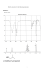### Special Situations Review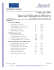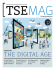### 2nd Edition November2013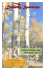### T - ENS de Lyon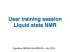### REXEL 3.3 - Università degli Studi di Napoli Federico II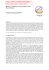### Flat Panel Display Cart V2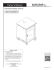### irvine spectrum® amenities - Irvine Company Offices for rent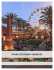### irvine spectrum® amenities - Irvine Company Offices for rent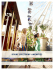### Application of the Public-Trust Doctrine and Principles of Natural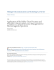### samena trends - SAMENA Telecommunications Council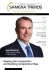### Wittgenstein and Qualia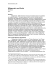### 0006609 - EN / Bilkent University